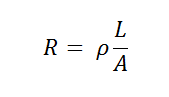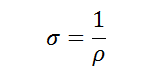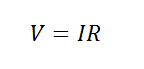# Resistivity: Is Copper the Best Metal Conductor?Where RIs resistance in ohms, L is the length of the wire in meters, A is the cross sectional area of the wire in square meters, and ρIs the electrical resistivity in ohm-meters.

Electrical ConductivityIs the inverse of resistivity: it’s the ability of a material to let current pass through. It is denoted by the Greek letter sigma, σ,And is measured in units of Siemens (S).In this experiment you will be able to find the resistivity and conductivity of the materials you test by using Ohm’s law, which states that VoltageIs proportional to the product of current and  resistance. The ammeter will help you measure the current that flows through the circuit, while the voltmeter will give you the voltage drop across the section you are testing.Where VIs the voltage, measured in volts, IIs the current, measured in Amperes, and RIs the resistance in ohms.

Problem: Observe the resistivity of different materials and material thicknesses and calculate electric conductivity.

Which material will be more resistive? Conductive?

Materials

• 9V battery
• 30cm of uninsulated copper wire (thinner gauge)
• 30cm of uninsulated copper wire (thicker gauge)
• 30cm of uninsulated iron wire (same gauge as the thinner gauge of copper wire)
• 30cm of uninsulated iron wire (same gauge as the thicker gauge of copper wire)
• Any other wires you would like to test
• Wire cutters
• Ammeter
• Voltmeter
• Ruler

Procedure

1. Attach the positive lead of the ammeter to the negative terminal of the 9V battery.
2. Attach the negative lead of the ammeter to one end of one of the wires.
3. Connect the other end of the wire to the positive terminal of the 9V battery.
4. Use the voltmeter to measure voltage drops across different lengths of the wire (start with 2cm, then measure 3cm, 4cm, etc.). Be sure that positive lead of the voltmeter touches the beginning of the wire.1. Record the current (from the ammeter) and the voltage drop (from the voltmeter) for each length of each tested wire.
2. Use Ohm’s law to determine the resistance and how length, gauge and material influences resistance.
3. Plot your results for each type of wire. Plot wire length (in meters) on the x-axis and resistance (in ohms) on the y-axis.
4. Calculate the resistivity using this formula:Where R Is resistance in Ohms ρIs the resistivity in ohm-meters LIs the length of the wire in meters and AIs the cross sectional area of the wire, in meters. *you can look up the cross sectional area for differeng gauges of wire online.

1. Use the resistivity, ρ, to calculate the electrical conductivity, σ.

Results

Thicker wires will have lower resistances, but longer wires will have higher resistances. Copper has a lower resistivity and is a better conductor of electricity than iron.

Why?

The resistance of a wire increases with length. Because resistance is the property of a material that resists electron flow, it makes sense that the more material you have (longer length) the more resistance you will have. Electrical resistivity, ρ, is a constant that is a property of the material, and it normalizes the resistance to the cross sectional area of the material the current is flowing through. The slope of the line on the plot of length vs. resistance is the electrical resistivity.

So is copper the best metal conductor? Copper is a better conductor than iron, which means current can flow easier (with less resistance) through copper. This is an inherent property of a material.

You can use Ohm’s law to calculate the resistance of the section you are measuring because the circuit is in Series, which means the current will be the same everywhere in the circuit.

Disclaimer and Safety Precautions

Education.com provides the Science Fair Project Ideas for informational purposes only. Education.com does not make any guarantee or representation regarding the Science Fair Project Ideas and is not responsible or liable for any loss or damage, directly or indirectly, caused by your use of such information. By accessing the Science Fair Project Ideas, you waive and renounce any claims against Education.com that arise thereof. In addition, your access to Education.com's website and Science Fair Project Ideas is covered by Education.com's Privacy Policy and site Terms of Use, which include limitations on Education.com's liability.

Warning is hereby given that not all Project Ideas are appropriate for all individuals or in all circumstances. Implementation of any Science Project Idea should be undertaken only in appropriate settings and with appropriate parental or other supervision. Reading and following the safety precautions of all materials used in a project is the sole responsibility of each individual. For further information, consult your state's handbook of Science Safety.

Create new collection

0

### New Collection>

0Items

What could we do to improve Education.com?